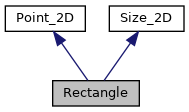PDS_JP2

# Rectangle Struct Reference

A Rectangle is a position with a size. More...

`#include <Dimensions.hh>`

Inheritance diagram for Rectangle:[legend]
Collaboration diagram for Rectangle:[legend]

List of all members.

## Public Member Functions

Rectangle ()
Constructs an empty rectangle.
Rectangle (const Point_2D &position, const Size_2D &size)
Constructs a rectangle from a position and a size.
Rectangle (const Size_2D &size)
Constructs a rectangle from a size at position 0,0.
Rectangle (const int x, const int y, const unsigned int width=0, const unsigned int height=0)
Constructs a rectangle from an x,y position and width,height size.
Rectangle (const Rectangle &rectangle)
Constructs a rectangle as a copy of another rectangle.
Rectangleoperator= (const Rectangle &rectangle)
Assign the position and size of another rectangle to this rectangle.
Point_2D position () const
Get the rectangle position.
Rectangleposition (const Point_2D &point)
Set the position of the rectangle.
Rectangleposition (const int x, const int y)
Set the x,y position of the rectangle.
Size_2D size () const
Get the rectangle size.
Rectanglesize (const Size_2D &size)
Set the size of the rectangle.
Rectanglesize (const unsigned int width, const unsigned int height)
Set the width,height of the rectangle.
Rectangleoperator&= (const Rectangle &rectangle)
Take the intersection with another rectangle.

## Detailed Description

A Rectangle is a position with a size.

Version:
1.7
See also:
Point_2D
Size_2D

## Constructor & Destructor Documentation

 Rectangle ( )

Constructs an empty rectangle.

The position is 0,0 and the size is 0,0.

 Rectangle ( const Point_2D & position, const Size_2D & size )

Constructs a rectangle from a position and a size.

Parameters:
 position A Point_2D. size A Size_2D.
 Rectangle ( const Size_2D & size )

Constructs a rectangle from a size at position 0,0.

Parameters:
 size A Size_2D.
 Rectangle ( const int x, const int y, const unsigned int width = `0`, const unsigned int height = `0` )

Constructs a rectangle from an x,y position and width,height size.

Parameters:
 x The horizontal (x-axis) position. y The vertical (y-axis) position. width The width of the rectangle size. height The height of the recangle size.
 Rectangle ( const Rectangle & rectangle )

Constructs a rectangle as a copy of another rectangle.

Parameters:
 rectangle A Rectangle to be copied.

## Member Function Documentation

 Rectangle & operator= ( const Rectangle & rectangle )

Assign the position and size of another rectangle to this rectangle.

Parameters:
 rectangle A Rectangle whose position and size are be assigned to this rectangle.
Returns:
This Rectangle.
 Point_2D position ( ) const` [inline]`

Get the rectangle position.

Returns:
A Point_2D with the rectangle position. N.B.: Changing this point will not change the position of the rectangle.

References Point_2D::Point_2D(), Point_2D::X, and Point_2D::Y.

 Rectangle& position ( const Point_2D & point ) ` [inline]`

Set the position of the rectangle.

Parameters:
 point A Point_2D for the position of the rectangle.
Returns:
This Rectangle.

References Point_2D::X, and Point_2D::Y.

 Rectangle& position ( const int x, const int y ) ` [inline]`

Set the x,y position of the rectangle.

Parameters:
 x The horizontal (x-axis) position. y The vertical (y-axis) position.
Returns:
This Rectangle.

References Point_2D::x(), Point_2D::X, Point_2D::y(), and Point_2D::Y.

 Size_2D size ( ) const` [inline]`

Get the rectangle size.

Returns:
A Size_2D with the rectangle size. N.B.: Changing this size will not change the size of the rectangle.

References Size_2D::Height, Size_2D::Size_2D(), and Size_2D::Width.

 Rectangle& size ( const Size_2D & size ) ` [inline]`

Set the size of the rectangle.

Parameters:
 size A Size_2D for the size of the rectangle.
Returns:
This Rectangle.

References Size_2D::Height, and Size_2D::Width.

 Rectangle& size ( const unsigned int width, const unsigned int height ) ` [inline]`

Set the width,height of the rectangle.

Parameters:
 width The width of the rectangle size. height The height of the recangle size.
Returns:
This Rectangle.

References Size_2D::height(), Size_2D::Height, Size_2D::width(), and Size_2D::Width.

 Rectangle & operator&= ( const Rectangle & rectangle )

Take the intersection with another rectangle.

Parameters:
 rectangle The Rectangle to intersect with this Rectangle.
Returns:
This Rectangle with its position and size set to the intersection with the other rectangle. If the rectangles do not intersect this will result in a rectangle with no area (width and height both zero) but the position will be unchanged.

The documentation for this struct was generated from the following files: